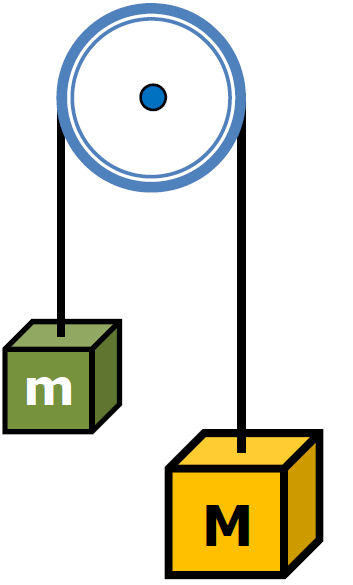﻿ HomeHomeAssuming a frictionless, massless pulley, ...

... determine the a of the block after the large block is releasedLet's make clockwise positive
Fnet = ma

[Mg - mg] = (M + m)ag(M - m) = a (m + M)

Only applies when surface frictionless

Finish This Sentence

According to Newton's 2nd LAw the acceleration of the 2 masses
comes from weight difference and .....Force of tension of the rope
on mass m, M?Rule - One Rope,
One Tension
Let's Make Clockwise PositiveΣFy = Ma

Mg - Tf = Ma

Tf  = Mg - Ma

Full ScreenPulley / Table